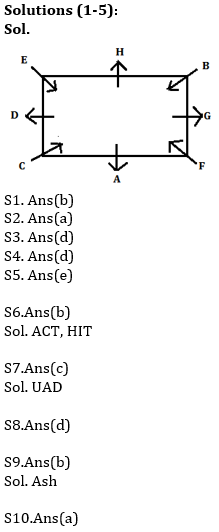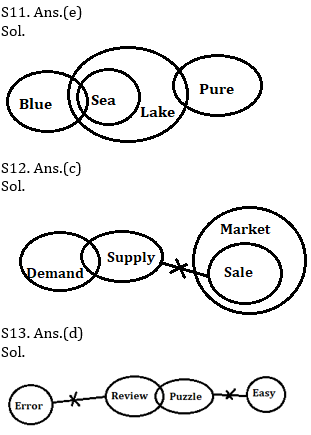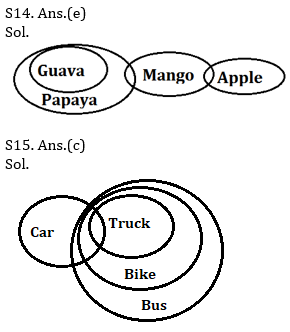Latest Banking jobs   »   Reasoning Ability Quiz For IBPS Clerk/NIACL...

# Reasoning Ability Quiz For IBPS Clerk/NIACL AO Prelims 2021- 27th August

Directions (1-5): Study the information carefully and answer the questions given below.
Eight persons i.e. A, B, C, D, E, F, G and H sit at square table. Four of them sit at corners and face inside. Remaining four sits at middle of the side and face outside. D sits second to the right of A. Two persons sit between D and F. G does not face D. H sits third to the right of F. B sits opposite to C. E sits second to the right of B.

Q1. Who among the following person sits second to the right of G?
(a) H
(b) A
(c) C
(d) E
(e) G

Q2. Who among the following person sits third to the right of C?
(a) G
(b) A
(c) F
(d) D
(e) H

Q3. How many persons sit between A and D when counted from left of A?
(a) One
(b) Two
(c) Three
(d) Five
(e) Four

Q4. Which of the following statement is true regarding E?
(a) E sits immediate right of B
(b) E sits second to the left of B
(c) None is true
(d) E sits at one of the corner
(e) E face outside

Q5. Four of the following five are alike in certain way based from a group, find the one that does not belong to that group?
(a) A-F
(b) B-H
(c) C-A
(d) D-E
(e) G-A

Directions (6-10): The following questions are based on the five words in each of the three letters given below.
KMD WUY ITH CSH TAC

Q6. If all the letters in each of the words are arranged in alphabetical order within the word, then how many meaningful words will be formed?
(a) One
(b) Two
(c) Three
(d) More than three
(e) None

Q7. If the first and last letter of each word replace by succeeding letter in English alphabet, then how many words have more than one vowel?
(a) Three
(b) Two
(c) One
(d) More than three
(e) None

Q8. If the first and last letters of each word are interchanged their position, then words are arranged in alphabetical order. Which of the following word is 3rd from right in new arrangement?
(a) ITH
(b) TAC
(c) KMD
(d) CSH
(e) None of these

Q9. If the first letter of each word replace by second preceding letter in English alphabetical series, then how many meaningful words will be formed?
(a) None
(b) One
(c) Two
(d) Three
(e) More than three

Q10. If all words are arranged in alphabetical order from left, after first letter of each word replaced by its reverse letter in alphabetical series, then which of the following word is 2nd from left?
(a) TAC
(b) KMD
(c) ITH
(d) CSH
(e) None of these

Directions (11-15): In each of the questions given below some statements are followed by some conclusions. You have to take the given statements to be true even if they seem to be at variance from commonly known facts. Read all the conclusions and then decide which of the given conclusions logically follows from the given statements disregarding commonly known facts.

Q11. Statements:
All Sea is lake
Some lake is Pure
Some sea is Blue
Conclusion:
I. Some pure is Blue
II. Some lake is blue is possibility
III. Some sea are pure
(a) Only I is true
(b) Only II is true
(c) Both I and II is true
(d) Only III is true
(e) None true

Q12. Statements:
Some Demand is supply
No supply is Sale
All sale is Market
Conclusion:
I. Some Demand is not Sale
II. Some Supply is market
III. All Demand can never be market
(a) Only II is true
(b) Both I and III is true
(c) Only I is true
(d) None follows
(e) Both I and II is true

Q13. Statements:
No Error is Review
Some review are puzzle
No puzzle is Easy
Conclusion:
I. Some puzzle are not error
II. No Error is Easy
III. Some Review is not Easy
(a) Both II and III is true
(b) Only III is true
(c) Both I and II is true
(d) Both I and III is true
(e) None of these

Q14. Statements:
All Guava are Papaya.
Some Papaya are Mango.
Some Apple are Mango.
Conclusions:
I. Some Apple are Papaya.
II. Some Mango are Guava.
III. Some Guava are Apple.
(a) Only I follows
(b) Only II follows
(c) Only I and III follow
(d) Only III follows
(e) None follows

Q15. Statements:
Some car are truck
All truck are Bike
All bike are Bus
Conclusions:
I. All car are Bike
II. All bike are truck.
III. Some car are not bike
(a) Only I follows
(b) Only II and III follow
(c) Either I and III follow
(d) Only I and II follow
(e) None of these

Solutions